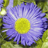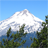# Modeling Automated Mileage Calculations:

285
3
10-06-2011 07:05 AMNew Contributor II
Hello, I am working on a two step process that would essentially calculate milieages and percentages between two datasets.  The goal is to automate a process to first calculate the total stream mileage (linear) within a Hydrologic Unit Code 12, and place the results within a predetermined field in the HUC12 (polygon).  The next step is to calculate the percentage of a specific stream type, using the totals generated from the first process.  Can this be done in a model environment, and what would be my simplest approach to automate the procedure to systematically process multiple HUC12s?

I've preprocessed the data to split streams at the HUC12 boundaries, and added the fields to house the newly created total and percentage values.  Thank you so much in advance...
Tags (3)
3 RepliesbyEsri Regular Contributor
Since you have the streams split by the HUC12 polygons, I assume they carry the polygon IDs. The Frequency tool (Analysis toolbox - Stat. toolset) can give you the sum of the shape_length of the streams based on the poly IDs; it can also give you the sum of the shape_length based on steam types. You can then Join the appropriate tables to get the percentage and total values calculated for the fields. Hope this helps.New Contributor II
Perfect.  Thank you Dan!  I was attempting to make it more complicated than needed.byEsri Regular Contributor
You are welcome, Mark! I am glad to help.

Cheers,